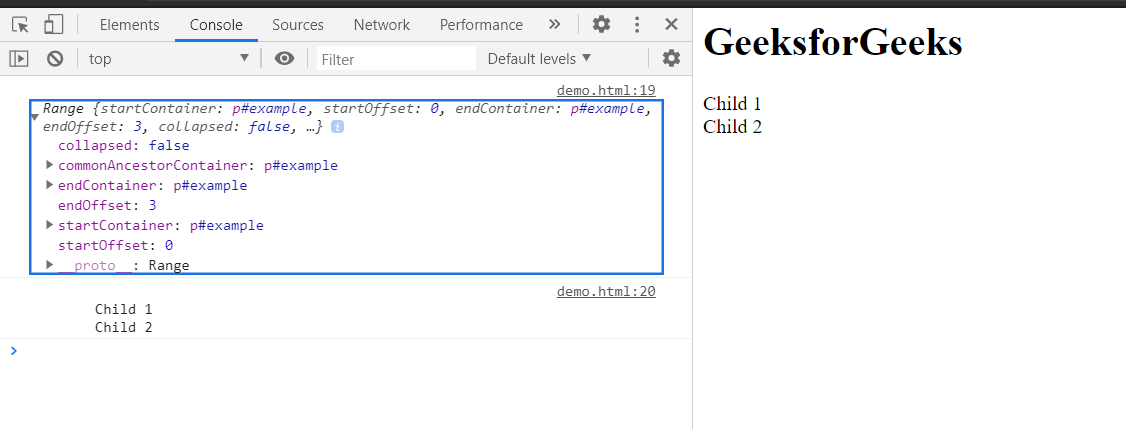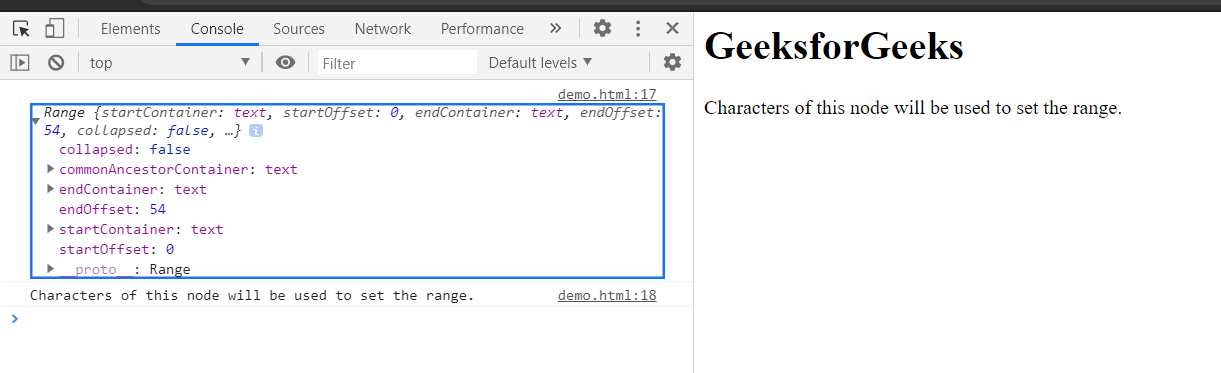# HTML DOM Range setEnd() Method

The setEnd() method is used to set the ending position of a Range.

The endNode can be used as a text node, child nodes etc. The endOffset can be the number of characters from the endNode or can be the number of child node of the endNode.

Syntax:

`range.setEnd(endNode, endOffset);`

Parameters: This method accepts two parameters as mentioned above and described below:

• endNode: The Node which is used to set the ending of the range.
• endOffset: This parameter is Offset index greater than or equal to zero representing the index for the ending of the Range.

Return Value: This method does not return any value.

Example 1: This example setting ending of range as child nodes of a parent node.

This example uses the setEnd() method to set the ending child node of the range. Here, we have used setStart() method to set the starting of the range to make up a range completely. For clarity of defined range, we have converted the selected range into text using toString() method.

## HTML

 ` ` `<``html``> ` ` `  `<``head``> ` `    ``<``title``> ` `        ``HTML DOM range setEnd() method ` `    `` ` ` ` ` `  `<``body``> ` `    ``<``h1``>GeeksforGeeks ` ` `  `    ``<``p` `id``=``"exampleNode"``> ` `        ``Child 1<``br``> ` `        ``Child 2<``br``> ` `    `` ` ` `  `    ``<``script``> ` `        ``const exampleNode = document ` `            ``.getElementById('exampleNode'); ` ` `  `        ``const range = document.createRange(); ` ` `  `        ``// 0th child gets at starting ` `        ``// of the range ` `        ``range.setStart(exampleNode, 0); ` ` `  `        ``// 3rd child gets at ending ` `        ``// of the range ` `        ``range.setEnd(exampleNode, 3); ` `        ``console.log(range); ` `        ``console.log(range.toString()); ` `    `` ` ` ` ` `  ` `

Output:  In console, the created range can be seen.Example 2: This example setting the ending of range as characters of a text node.

## HTML

 ` ` `<``html``> ` ` `  `<``head``> ` `    ``<``title``> ` `        ``HTML DOM range setEnd() method ` `    `` ` ` ` ` `  `<``body``> ` `    ``<``h1``>GeeksforGeeks ` ` `  `    ``<``p` `id``=``"exampleNode"``> ` `        ``Characters of this node will  ` `        ``be used to set the range. ` `    `` ` ` `  `    ``<``script``> ` `        ``const exampleNode = document ` `            ``.getElementById('exampleNode'); ` ` `  `        ``const textNode = exampleNode.childNodes; ` `        ``const range = document.createRange(); ` ` `  `        ``// Starting of range will be 0th character ` `        ``range.setStart(textNode, 0); ` ` `  `        ``// Ending of range will be 54th character ` `        ``range.setEnd(textNode, 54); ` `        ``console.log(range); ` `        ``console.log(range.toString()) ` `    `` ` ` ` ` `  ` `

Output: In console, the created range can be seen.Supported Browsers:

• Edge
• Firefox
• Safari
• OperaMy Personal Notes arrow_drop_upCheck out this Author's contributed articles.

If you like GeeksforGeeks and would like to contribute, you can also write an article using contribute.geeksforgeeks.org or mail your article to contribute@geeksforgeeks.org. See your article appearing on the GeeksforGeeks main page and help other Geeks.

Please Improve this article if you find anything incorrect by clicking on the "Improve Article" button below.

Article Tags :
Practice Tags :

Be the First to upvote.

Please write to us at contribute@geeksforgeeks.org to report any issue with the above content.